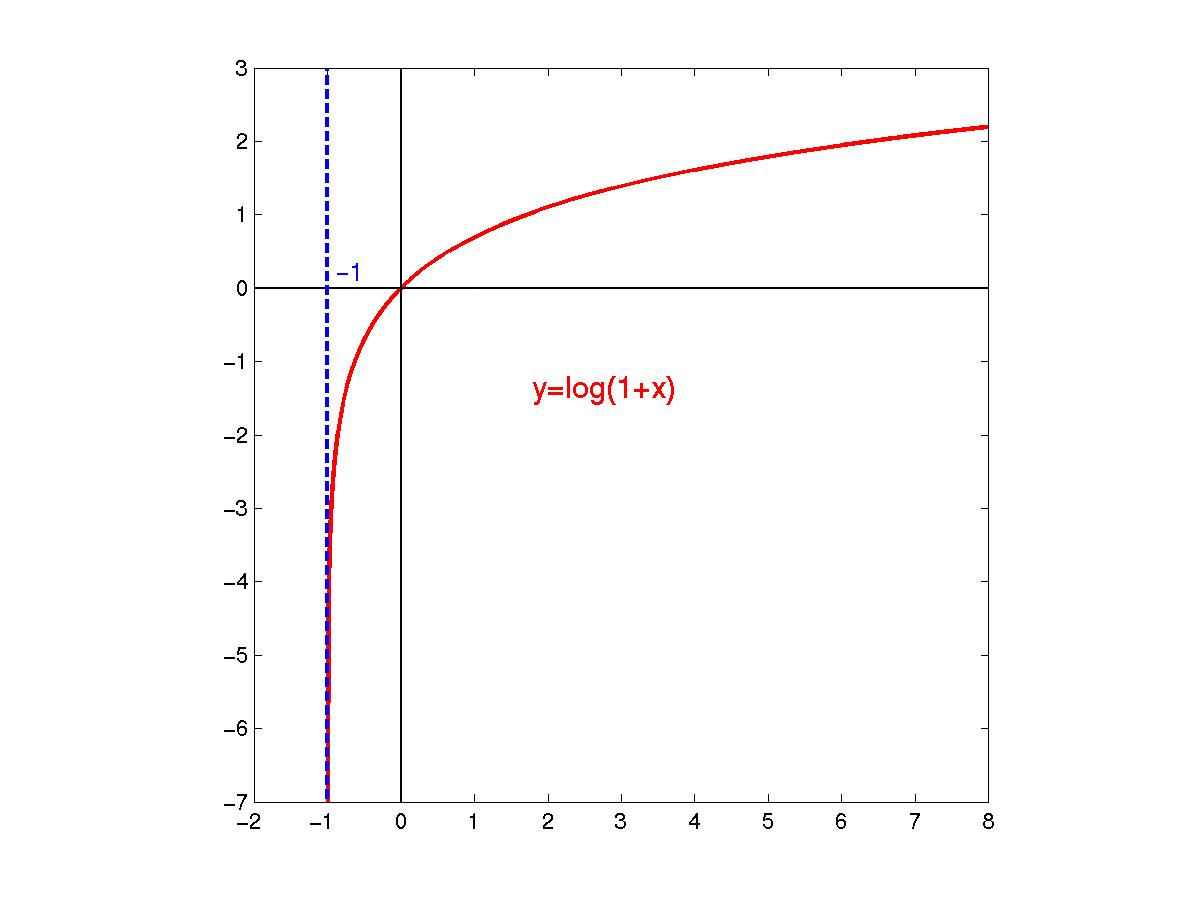# Math.数学公式合集()

WARNING

## Math.exp()#

### 语法#

exp(x: number): number;

### 示例#

Math.exp(-1) // 0.36787944117144233

## Math.log()#

### 语法#

log(x: number): number;

### 描述#

• 真数为负数时返回 NaN

• 真数为 0 时返回 -Infinity

### 示例#

Math.log(-1) // NaN
Math.log(0) // -Infinity
Math.log(1) // 0
Math.log(10) // 2.302585092994046

function getBaseLog(x, y) {
return Math.log(y) / Math.log(x)
}
// IEEE-754 精度问题
getBaseLog(10, 1000) // 2.9999999999999996

## Math.log10()#

### 语法#

log10(x: number): number;

### 示例#

Math.log10(1) // 0
Math.log10(100) // 2

## Math.log1p()#

### 语法#

log1p(x: number): number;

### 描述#### 示例#

Math.log1p(Math.E - 1) // 1
Math.log1p(Math.pow(Math.E, 2) - 1) // 2.1269280110429722

## Math.log2()#

### 语法#

log2(x: number): number;

### 示例#

Math.log2(1024) // 10
Math.log2(1) // 0

## Math.sqrt()#

### 语法#

sqrt(x: number): number;

### 示例#

Math.sqrt(1) // 1
Math.sqrt(0) // 0
Math.sqrt(-1) // NaN
Math.sqrt(1024) // 32

## Math.cbrt()#

### 语法#

cbrt(x: number): number;

### 示例#

Math.cbrt(1) // 1
Math.cbrt(0) // 0
Math.cbrt(-1) // -1
Math.cbrt(512) // 8

## Math.pow()#

### 语法#

pow(x: number, y: number): number;

### 示例#

Math.pow(2, 4) // 16
Math.pow() // NaN
Math.pow(2) // NaN

## Math.hypot()#

### 语法#

hypot(...values: number[]): number;

### 描述#

• 当不传入参数时返回 0 （特例）

• 可以传入一个负数，因为平方时被转换成了正数

### 示例#

Math.hypot(3, -4) // 5
Math.hypot() // 0

## Math.expm1()#

### 语法#

expm1(x: number): number;

### 示例#

Math.expm1(0) // 0
Math.expm1(1) // 1.718281828459045
Last updated on by YanceyOfficial